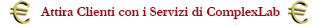# STGraph : a simple simulator for complex dynamic systems

STGraph is a simulator for dynamic systems modeled according to the state-variable approach to System Theory in the synchronous, discrete time case. Systems are qualitatively modeled as directed graphs, whose nodes and edges represent respectively variables and dependency relations in variable definition.

An introduction to STGraph

Luca Mari, Version 4.11.07

The latest version of this "Introduction to STGraph" document can be freely downloaded from the file in next right column otherwise from the site: http://www.liuc.it/persone/lmari/stgraph/app/intro.pdf

Here below you'll find some excerpts from such document:

STGraph is a simulator for dynamic systems modeled according to the state-variable approach to System.

Theory in the synchronous, discrete-time case.

Systems are qualitatively modeled in STGraph as directed graphs, whose nodes and arrows (i.e., directed edges) represent respectively variables and dependence relations in variable definition.

STGraph is a simulator for dynamic systems modeled according to the state-variable approach to System.

Expression Language

The language by which the expressions are defined, here called STEL (STgraph Expression Language), is a functional one, so that each node expression always produces one (and only one) value as result of its evaluation, no explicit variable assignment is allowed and no side effects can be generated in the evaluation. STEL is a weakly typed language (i.e., the variable type is automatically assigned by the system), with double as the basic data type, also interpreted as integer or boolean when required. Each variable can be:

• a scalar, written in decimal (e.g., 1.23) or scientific (e.g., 1.23E2) format; all strictly positive values are dealt with as the boolean 'true', and zero and negative values as the boolean 'false';
• a (column) vector, i.e., dynamic 1D array of scalars, written, e.g., as [1,2,3] to define the vector of the three scalars 1, 2, and 3;
• a matrix, i.e., dynamic 2D array of scalars, written, e.g., as [[1,2,3],[4,5,6]] to define the matrix of 2 rows and 3 columns whose element in first row and first column is the scalar1, the element in first row and second column is the scalar 2, and so on.

Logic of execution

Each node can be used to define an arbitrary number of other nodes, i.e., an arbitrary number of outgoing arrows can be drawn from each node. Conversely, each node can be defined by an arbitrary number of other nodes, i.e., an arbitrary number of incoming arrows can be drawn to each node. The network of the arrows in the graph implicitly defines the sequence of evaluation.

Graph topology

The node types also constrain the graph topology, and specifically the possibility of introducing loops (acyclic graphs are always topologically correct), according to the following general rule:

• any loop must include at least one state node also taking into account that from the point of view of this rule state nodes with distinct output are considered equivalent to auxiliary nodes.

Submodels

Together with the listed node types, STGraph includes the submodel node type, by which each previously generated model can be embedded as a submodel in a model. An embedded submodel is accessible to its supermodel only through its input and output nodes, according to the following logic:

• the input nodes of a submodel are automatically exposed to the supermodel via the submodel node configuration dialog. Hence, the value of each input node of a submodel can be either left unassigned, and in this case its value is computed by its output function, or can be assigned by the supermodel;
• the output nodes of a submodel are automatically exposed to the supermodel as multiple node outputs, each of them accessible with the syntax submodelnode.outputnode.

The latest version of this "Introduction to STGraph" document can be freely downloaded from the file in next right column otherwise from the site: http://www.liuc.it/persone/lmari/stgraph/app/intro.pdf

For information and consultancy ( Per informazioni e consulenze ):

• Email: lmari@liuc.it
• Tel. : +39 0331 572228
• Tel. : +39 0331 572228
• Tel. : +39 0331 572228
##### SCARICA GLI ALLEGATI
archiviato sotto:

### Luca Mari

Dello stesso autore
Complessità dall’analisi di (modelli di) sistemi dinamici: qualche esempio (seconda parte)

Questo secondo articolo pone le questioni: a quali conseguenze conduce la trasformazione da tempo continuo a tempo discreto? Sotto quali condizioni la previsione rimane di qualità sufficiente? L�...

Complessità dall’analisi di (modelli di) sistemi dinamici: qualche esempio

L'articolo pone il meta-problema "data una qualsiasi equazione a tempo locale, è sempre possibile trovare la sua corrispondente a tempo globale?", partendo dal pratico e quotidiano esempio di un c...

Può l'approccio sistemico aiutarci a gestire la complessità?

Un approccio demistificatore alla complessità, con gli occhi di un epistemologo, per comprendere "come comprendere" la complessità...

×